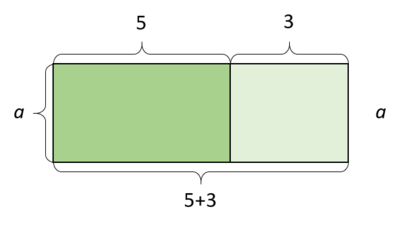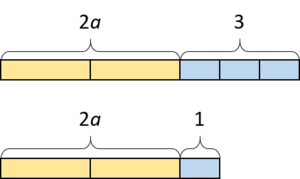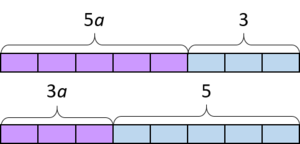# All, Some, or None?

Alignments to Content Standards: 6.EE.B

For each equation, explain whether it's true for all, some, or no values of $a$. Explain how you know.

1. $\quad(3+5)a=5a+3a$
2. $\quad 2a+1=2a+3$
3. $\quad 5a+3=3a+5$

## IM Commentary

In earlier grades, students write and evaluate numerical expressions and equations to represent operations with numbers. Their experience with the meaning of addition, subtraction, multiplication, and division as well as the properties of operations prepares them to understand algebra as “generalized arithmetic.” Since numerical expressions can only have one value, an equation with only numbers, such as $2^3 = 9-1$, is either true or false, depending on whether the expression on the left has the same value as the expression on the right. On the other hand, there is an important distinction between being equal and being equivalent for algebraic expressions. For example, the expressions $3x + 5$ and $5x + 3$ are equal when $x = 1$, but they are not equivalent. The expressions $x(2 + 3)$ and $3x + 2x$ are equivalent because they are equal no matter the value of $x$. So an algebriac equation is sometimes viewed as an implict question: "For what values of the variable is the equation true?"

This is a rich discussion question that spotlights the different possibilities for an equation when a variable is involved. It’s important to encourage students to understand what a solution to an equation is and what it means to solve an equation before they learn general and efficient procedures. Students should think about what an equation is saying and what solution(s) are reasonable before solving it; for example when trying to solve $10=2x$, students think about what number times $2$ would equal $10$. Throughout grade 6, students should see a variety of equations, some of which have only one solution, some which are identities, and some with no solution; these states correspond with being true for one value, all values, and no values of a variable. Sometimes when algebraic equations are introduced in grade 6, students are just asked to write an equation for a problem that they could easily solve numerically. This task represents a way to have a meaningful conversation about equations as algebraic objects with potentially different characteristics.

Considering all three equations at once or in the same class period might be a too much for one day; the discussion can be spread out over three different class periods. If a class has a "number talk" or "algebra talk" routine, these items might fit nicely in that structure.

These equations are somewhat complicated relative to the other work of sixth grade, so if appropriate, a teacher may decide to replace or preface this task with a task that asks the same question ("all, some, or none") but uses simpler equations. For example, you could use $20 \cdot x \div 4 = 5x$ for the identity, $x=x+2$ which is true for no values of $x$, and $12=3x$ which is only true for one value of $x$.

## Solutions

Solution: Solution based on testing values

$(3+5)a=5a+3a$ This equation is true for all values of $a$. We can't prove it by testing different values of $a$, but we can gather as much evidence as we want. Here are some different values of $a$:

 $a$ Sub into $(3+5)a=5a+3a$ Calculations Outcome 0 $(3+5)\cdot0=5\cdot0+3\cdot0$ $8\cdot0=0+0$ $0=0$ 1 $(3+5)\cdot1=5\cdot1+3\cdot1$ $8\cdot1=5+3$ $8=8$ 40 $(3+5)\cdot40=5\cdot40+3\cdot40$ $8\cdot40=200+120$ $320=320$ $\frac{1}{16}$ $(3+5)\cdot\frac{1}{16}=5\cdot\frac{1}{16}+3\cdot\frac{1}{16}$ $8\cdot\frac{1}{16}=\frac{5}{16}+\frac{3}{16}$ $\frac{8}{16}=\frac{8}{16}$

$2a+1=2a+3$ This equation is never true for any value of $a$. We can't prove it by testing different values of $a$, but we can gather as much evidence as we want. Here are some different values of $a$:

 $a$ Sub into $2a+1=2a+3$ Calculations Outcome 0 $2\cdot0+1=2\cdot0+3$ $0+1=0+3$ $1=3$ 1 $2\cdot1+1=2\cdot1+3$ $2+1=2+3$ $3=5$ 40 $2\cdot40+1=2\cdot40+3$ $80+1=80+3$ $81=83$ $\frac{1}{16}$ $2\cdot\frac{1}{16}+1=2\cdot\frac{1}{16}+3$ $\frac{2}{16}+1=\frac{2}{16}+3$ $\frac{18}{16}=\frac{50}{16}$

$5a+3=3a+5$ This equation is true for some values (actually, only one value) of $a$ but not others. We can prove it by testing values if we show one value that makes the equation true and one value that makes the equation false.

 $a$ Sub into $5a+3=3a+5$ Calculations Outcome 1 $5\cdot1+3=3\cdot1+5$ $5+3=3+5$ $8=8$ 2 $5\cdot2+3=3\cdot2+5$ $10+3=6+5$ $13=11$

Solution: Solution based on algebraic structure

1. $(3+5)a=5a+3a$. This equation is true for all values of $a$. We can use algebraic structure to rewrite each side as identical expressions. There are many ways to do this; here are two examples:
• The left side is something-in-parentheses times $a$, and the something-in-parentheses is equivalent to $8$, so we can rewrite the left side as $8a$. On the right side, we are summing 5 $a$'s and 3 $a$'s, so all together we have $8a$.
• By the distributive property, we can rewrite the left side as $3a+5a$. We can then use the commutative property to rewrite the left side again as $5a+3a$. It is now identical to the right side.
2. $2a+1=2a+3$. This equation is never true for any value of $a$. Each side contains the term $2a$. On the left side, $1$ is added to the $2a$ and on the right side, $3$ is added to the $2a$. In order for this equation to be true, $1$ would have to equal $3$.
3. $5a+3=3a+5$. This statement is true for exactly one value of $a$. We can use a logical chain of reasoning to figure out that the value that would make the equation true is $1$. For example, we might rewrite the equation as something equivalent like $(3a+3)+2a=(3a+3)+2$, reason that if this is true then $2a=2$, and the value of $a$ we could multiply by $2$ and get a $2$ is $1$.

Solution: Solution based on diagrams

Even if students do not think to represent these equations with diagrams themselves, the teacher might still show these to reinforce the conclusions students have made through numeric and algebraic reasoning:

1. $(5 + 3)a = 3a + 5a$ for any value of $a$.This diagram can be viewed as a single rectangle with side lengths $a$ and $5+3$, and so represents $a(5+3)$. It can also be viewed as two smaller rectangles, one with side-lengths $a$ and 3 and area $3a$, and the other with side-lengths $a$ and 5 and area $5a$. Since the area is the same either way, these two values must be equal for any positive value of $a$.

2. $2a+3$ never equals $2a+1$.This diagram shows that no matter what the value of $a$ is, these two expressions can never be equal.
3. $5a+3=3a+5$ if $a=1$.This diagram is hard to draw correctly without knowing the answer, but a dynamic version of it can help.

Here is an alternate dynamic version of the first diagram:

Here is a dynamic version of the second diagram: